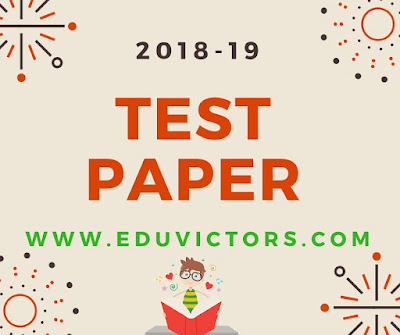## CBSE Class 11 - Physics Sample Question Paper - (2018-19)

Physics is a study of basic laws of nature and their manifestation in different natural phenomena. Physics is the study of physical world and matter and its motion through space and time, along with related concepts such as energy and force. The class 11 syllabus provides logical sequencing of the ‘Units’ of the subject matter with proper placement of concepts with their linkages for better understanding.

Here follows the class 11 Half Year Physics sample question paper to assess your knowledge.

Physics Syllabus (2017-18)
Simple Physics Quiz
Ch2 Range of Measurements
Ch2 -  Error Analysis
Ch2 - Dimension Analysis
Ch2 - Units and Measurements (MCQs)
Physical Constants

Types of Vectors

Ch3 Motion in Straight Line - 1
Ch3 Motion in Straight Line - 2

Motion In a Straight Line (Revision Test)

Projectile Motion (Assignment Sheet)

Work Energy Power (Revision Test)
Work Energy Power (Assignment -1)

Laws Of Motion (Revision Test)

Gravitation - Understanding Mass and Weight
Gravitation - Kepler's Laws

Centre Of Mass and Rotational Motion (Assignment)